#### Analysis with Surface Trend Removal

You can use a SAS/STAT predictive modeling procedure to extract surface trends from your original data. If your goal is spatial prediction, you can continue processing the detrended data for the prediction tasks, and at the end you can reinstate the trend at the prediction locations to report your analysis results.

In general, the exact form of the trend is unknown, as discussed in the section Empirical Semivariograms and Surface Trends. In this case, the spatial distribution of the measurements shown in Output 102.2.1 suggests that you can use a quadratic model to describe the surface trend like the one that follows: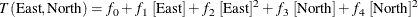The following statements show how to invoke the GLM procedure for your ozone data and how to extract the preceding trend from them:

proc glm data=ozoneSet plots=none;
model ozone = East East*East North North*North;
output out=gmout predicted=pred residual=ResidualOzone;
run;


Among other output, PROC GLM produces estimates for the parameters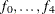in the preceding trend model. Output 102.2.4 shows the table with the parameter estimates. In this table, the coefficient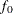corresponds to the intercept estimate, and the rest of the coefficients correspond to their matching variables; for example, the estimate in the line of East*East refers to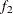in the preceding model. For more information about the syntax and the PROC GLM output, see Chapter 42: The GLM Procedure.

Output 102.2.4: Parameter Estimates for the Surface Trend Model

 Semivariogram Analysis in Anisotropic Case With Trend Removal

The GLM Procedure

Dependent Variable: Ozone

Parameter Estimate Standard Error t Value Pr > |t|
Intercept 270.6798273 0.40595731 666.77 <.0001
East 0.0065148 0.01360281 0.48 0.6323
East*East 0.0010726 0.00012987 8.26 <.0001
North -0.0369159 0.01297491 -2.85 0.0047
North*North 0.0035587 0.00012659 28.11 <.0001

The detrending process leaves you with the GMOUT data set, which contains the ResidualOzone data residuals. This time you run PROC VARIOGRAM again with the NOVARIOGRAM option to inspect the detrended residuals, and with a request only for the observations plot, as follows:

proc variogram data=gmout plots(only)=observ;
compute novariogram nhc=35;
coord xc=East yc=North;
var ResidualOzone;
run;


The requested observations plot is shown in Output 102.2.5.

Output 102.2.5: Ozone Residual Observation Data Scatter Plot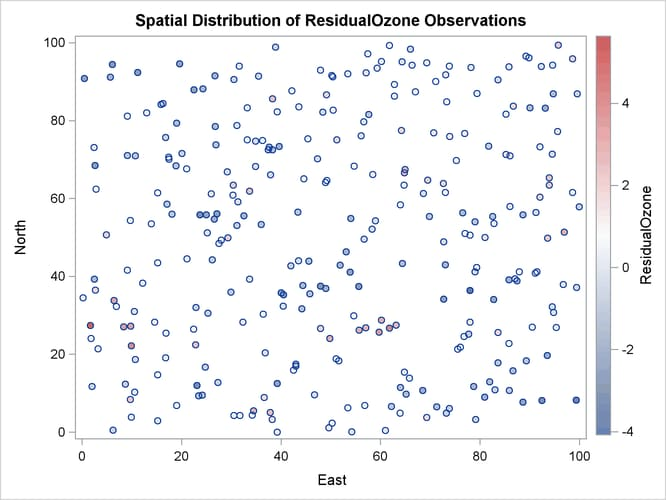Before you proceed with the empirical semivariogram computation and model fitting, examine your data for anisotropy. This investigation is necessary to portray the spatial structure of your SRF accurately. If anisotropy exists, it manifests itself as different ranges or sills or both for the empirical semivariograms in different directions.

You want detail in your analysis, so you ask for the empirical semivariance in 12 directions by specifying NDIRECTIONS=12. Based on the NDIRECTIONS= option, empirical semivariograms are produced in increments of the base angle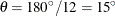.

You also choose ANGLETOLERANCE=22.5 and BANDWIDTH=20. A different choice of values produces different empirical semivariograms, because these options can regulate the number of pairs that are included in a class. Avoid assigning values that are too small to these parameters so that you can allow for an adequate number of point pairs per class. At the same time, the higher the values of these parameters are, the more data pairs that come from closely neighboring directions are included in each lag. Therefore, values for the ANGLETOLERANCE= and BANDWIDTH= options that are too high pose a risk of losing information along the particular direction. The side effect occurs because you incorporate data pairs from a broader spectrum of angles; thus, you potentially amplify weaker anisotropy or weaken stronger anisotropy, as noted in the section Angle Classification. You can experiment with different ANGLETOLERANCE= and BANDWIDTH= values to reach this balance with your data, if necessary.

With the following statements you ask to display only the SEMIVAR plots in the specified number of directions. Multiple empirical semivariograms are placed by default in panels, as Output 102.2.6 shows. If you want an individual plot for each angle, then you need to further specify the plot option SEMIVAR(UNPACK).

proc variogram data=gmout plot(only)=semivar;
compute lagd=4 maxlag=16 ndir=12 atol=22.5 bandw=20;
coord xc=East yc=North;
var ResidualOzone;
run;


Output 102.2.6: Ozone Empirical Semivariograms with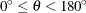and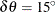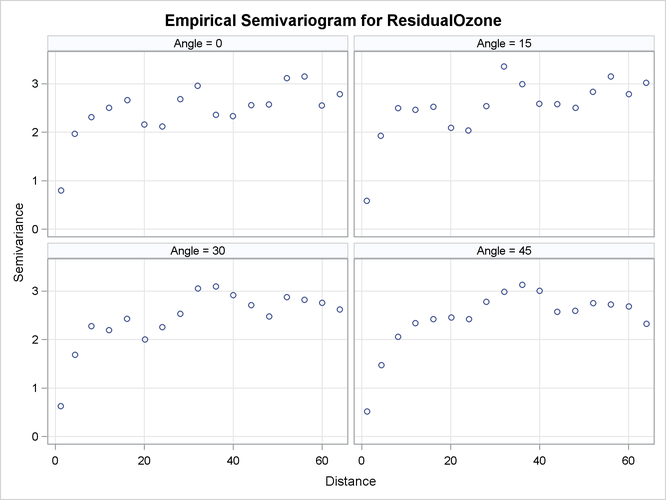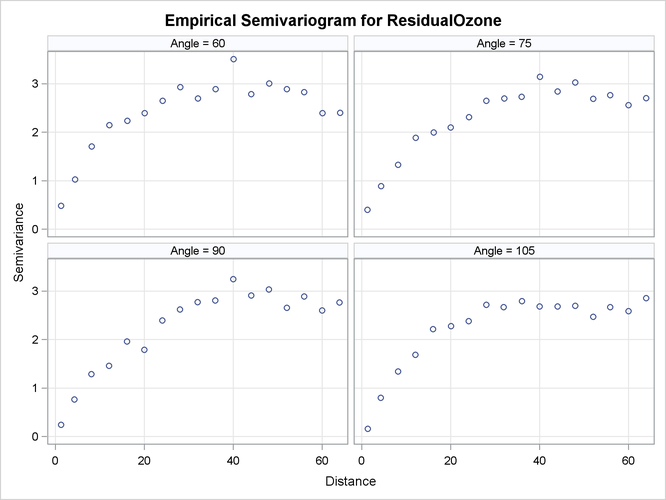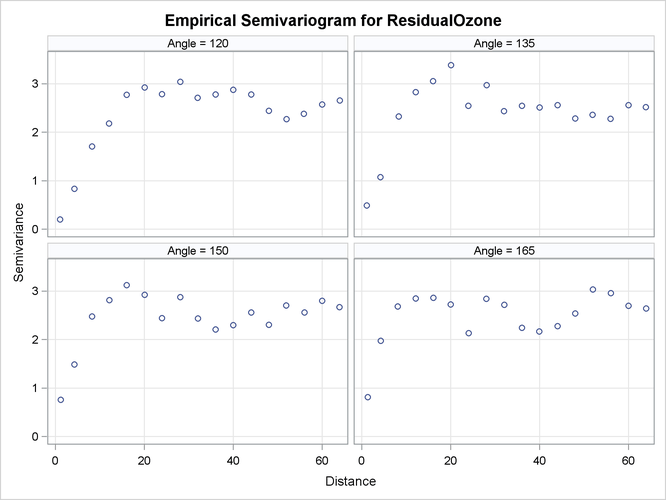The panels in Output 102.2.6 suggest that in some of the directions, such as for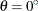, the directional plots tend to exhibit a somewhat noisy structure. This behavior can be due to the pairs distribution across the particular direction. Specifically, based on the LAGDISTANCE= choice there might be insufficient pairs present in a class. Also, depending on the ANGLETOLERANCE= and BANDWIDTH= values, too many pairs might be considered from neighboring angles that potentially follow a modified structure. These are factors that can increase the variability in the semivariance estimate. A different explanation might lie in the existence of outliers in the data set; this aspect is further explored in A Box Plot of the Square Root Difference Cloud.

This behavior is relatively mild here and should not obstruct your goal to study anisotropy in your data. You can also perform individual computations in any direction. By doing so, you can fine-tune the computation parameters and attempt to obtain smoother estimates of the sample semivariance.

Further in this study, the directional plots in Output 102.2.6 suggest that during shifting fromto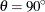, the empirical semivariogram range increases. Beyond the angle, the range starts decreasing again until the whole circle is traversed at 180and small range values are encountered around the N–S direction at. The sill seems to remain overall the same. This analysis suggests the presence of anisotropy in the ozone concentrations, with the major axis oriented at aboutand the minor axis situated perpendicular to the major axis at.

The multidirectional analysis requires that for a given LAGDISTANCE= you also specify a MAXLAGS= value. Since the ozone correlation range might be unknown (as assumed here), you can apply the rule of thumb that suggests use of the half-extreme data distance in the direction of interest, as explained in the section Spatial Extent of the Empirical Semivariogram. Following the information displayed in Output 102.2.3, for different directions this distance varies between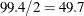and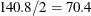km. In turn, the pairwise distances table in Output 102.2.2 indicates that within this range of distances you can specify MAXLAGS= to be between 12 and 17 lags. In this example you specify MAXLAGS=16.

At this point you are ready to continue with fitting theoretical semivariogram models to the empirical semivariogram in the selected directions ofand. By trying out different models, you see that an exponential one is suitable for your empirical data: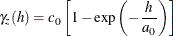For the purpose of the present example, it is reasonable to assume a constant nugget effect equal to zero, based on the empirical semivariograms shown in Output 102.2.6. The same output suggests that the model scale is likely to be above 2, and that the range might be relatively small in. You specify the PARMS statement to set initial values for the exponential model parameters and account for these considerations.

In particular, you assign an initial value of zero to the nugget effect. Then you request a grid search for the range and scale parameters, so that the optimal initial values set is selected for the parameter estimation in each of the two anglesand. By inspecting the empirical semivariograms in Output 102.2.6, you specify the value list 2, 2.5, and 3 for the scale, and the values from 5 to 25 with a step of 10 for the range. In addition, you specify the parameter 1 in the HOLD= option to designate the nugget effect parameter as a constant. According to these specifications, you use the following statements:

proc variogram data=gmout plot(only)=fit;
compute lagd=4 maxlag=16;
directions 0(22.5,10) 90(22.5,10);
coord xc=East yc=North;
model form=exp;
parms (0.) (2 to 3 by 0.5) (5 to 25 by 10) / hold=(1);
var ResidualOzone;
run;

ods graphics off;


The VARIOGRAM procedure repeats the fitting process for each one of the selected directions. First, inthe parameter search table in Output 102.2.7 shows you which value combinations are tested initially to choose the one that gives the lowest objective function value.

Output 102.2.7: Parameter Search for the Selected DirectionSemivariogram Analysis in Anisotropic Case With Trend Removal

The VARIOGRAM Procedure
Dependent Variable: ResidualOzone
Angle: 0
Current Model: Exponential

Parameter Search
Set Nugget Scale Range Objective
Function
1 0 2 5 391.06593
2 0 2 15 1740.0
3 0 2 25 5167.5
4 0 2.5 5 64.86565
5 0 2.5 15 664.03665
6 0 2.5 25 2480.5
7 0 3 5 72.86743
8 0 3 15 305.53306
9 0 3 25 1305.0

From this search, the combination of scale equal to 2.5 and a range of size 5 is passed as initial values to the model fitting process. This result is reflected in the model information table shown in Output 102.2.8.

Output 102.2.8: Model Initial Values for the Selected DirectionModel Information
Parameter Initial
Value
Status
Nugget 0 Fixed
Scale 2.5000
Range 5.0000

Fitting is successful, and among the output objects you can see the estimated parameters and the fit summary tables for the directionin Output 102.2.9.

Output 102.2.9: Weighted Least Squares Fitting Parameter Estimates and Summary for the Selected DirectionParameter Estimates
Parameter Estimate Approx
Std Error
DF t Value Approx
Pr > |t|
Scale 2.6657 0.03830 15 69.60 <.0001
Range 3.7277 0.5609 15 6.65 <.0001

Fit Summary
Model Weighted
SSE
AIC
Exp 43.35103 19.91399

A corresponding parameter search takes place for the direction. The respective table and the choice of initial values for fitting in the directionare shown in Output 102.2.10.

Output 102.2.10: Parameter Search and Model Initial Values for the Selected DirectionParameter Search
Set Nugget Scale Range Objective
Function
1 0 2 5 302.54551
2 0 2 15 635.93338
3 0 2 25 1996.0
4 0 2.5 5 95.09939
5 0 2.5 15 104.56776
6 0 2.5 25 662.06813
7 0 3 5 155.50670
8 0 3 15 20.48482
9 0 3 25 190.30599

Model Information
Parameter Initial
Value
Status
Nugget 0 Fixed
Scale 3.0000
Range 15.0000

Output 102.2.11 displays the estimated parameters and the fit summary for the direction.

Output 102.2.11: Weighted Least Squares Fitting Parameter Estimates and Summary for the Selected DirectionParameter Estimates
Parameter Estimate Approx
Std Error
DF t Value Approx
Pr > |t|
Scale 2.9199 0.07007 15 41.67 <.0001
Range 14.7576 0.9530 15 15.49 <.0001

Fit Summary
Model Weighted
SSE
AIC
Exp 19.12246 6.00005

The fitted and empirical semivariograms for the selected directions are displayed in the panel of Output 102.2.12.

Output 102.2.12: Fitted Theoretical and Empirical Semivariogram for the Ozone Data in theandDirections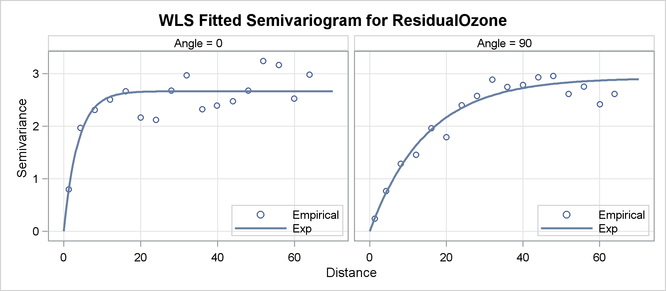Conclusively, your semivariogram analysis on the detrended ozone data suggests that the ozone SRF exhibits anisotropy in the perpendicular directions of N–S () and E–W ().

The sills in the two directions of anisotropy are similar in size. By inspecting again the empirical semivariograms in Output 102.2.6, you could make the reasonable assumption that you have a case of geometric anisotropy, where the range in the major axis is about 4.5 times larger than the minor axis range. If you would like to use these PROC VARIOGRAM results for predictions, then you would need to specify a single scale value for the geometric anisotropy sill. In this case you could choose an arbitrary value for the constant scale from the narrow interval formed by the estimated scales in the previous results. For example, you can specify the PARMS statement modified as shown in the following statement to approximate a common scale for the semivariance in all directions:

parms (0.) (2.7) (5 to 25 by 10) / hold=(1,2);


As an alternative, you can use PROC VARIOGRAM to fit an exponential model to all different angles examined in this example, and then select the constant scale value to be the mean of the scales across all directions.